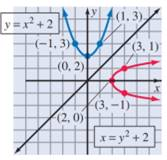# Further Investigations The points ( x , y ) and ( y , x ) are mirror images of each other across the line y = x . Therefore, by interchanging x and y in the equation y = a x 2 + b x + c , we obtain the equation of its mirror image across the line y = x ; namely, x = a y 2 + b y + c . Thus to graph x = y 2 + 2 , we can first graph y = x 2 + 2 and then reflect it across the line y = x , as indicated in Figure 8.22. Graph each of the following parabolas. x = y 2 x = − y 2 x = y 2 − 1 x = − y 2 + 3 x = − 2 y 2 x = 3 y 2 x = y 2 + 4 y + 7 x = y 2 − 2 y − 3 Figure 8.22### Intermediate Algebra

10th Edition
Jerome E. Kaufmann + 1 other
Publisher: Cengage Learning
ISBN: 9781285195728

#### Solutions

Chapter
Section### Intermediate Algebra

10th Edition
Jerome E. Kaufmann + 1 other
Publisher: Cengage Learning
ISBN: 9781285195728
Chapter 8.2, Problem 62PS
Textbook Problem
1 views

## Further InvestigationsThe points ( x , y ) and ( y , x ) are mirror images of each other across the line y = x . Therefore, by interchanging x and y in the equation y = a x 2 + b x + c , we obtain the equation of its mirror image across the line y = x ; namely, x = a y 2 + b y + c . Thus to graph x = y 2 + 2 , we can first graph y = x 2 + 2 and then reflect it across the line y = x , as indicated in Figure 8.22.Graph each of the following parabolas. x = y 2 x = − y 2 x = y 2 − 1 x = − y 2 + 3 x = − 2 y 2 x = 3 y 2 x = y 2 + 4 y + 7 x = y 2 − 2 y − 3Figure 8.22

To determine

(a)

To graph:

The given parabola x=y2.

### Explanation of Solution

Given:

The points (x,y) and (y,x) are mirror images of each other across the line y=x. Therefore, by interchanging x and y in the equation y=ax2+bx+c, obtain the equation of its mirror image across the line y=x; namely, x=ay2+by+c. Thus to graph x=y2+2, first graph y=x2+2 and then reflect it across the line y=x, as indicated in Figure (1)

To determine

(b)

To graph:

The given parabola x=y2.

To determine

(c)

To graph:

The given parabola x=y21.

To determine

(d)

To graph:

The given parabola x=y2+3.

To determine

(e)

To graph:

The given parabola x=2y2.

To determine

(f)

To graph:

The given parabola x=3y2.

To determine

(g)

To graph:

The given parabola x=y2+4y+7.

To determine

(h)

To graph:

The given parabola x=y22y3.

### Still sussing out bartleby?

Check out a sample textbook solution.

See a sample solution

#### The Solution to Your Study Problems

Bartleby provides explanations to thousands of textbook problems written by our experts, many with advanced degrees!

Get Started

Find more solutions based on key concepts
Evaluate the integral: 04x2x2ydy

Calculus: Early Transcendental Functions

True or False: n=21(lnn)n converges.

Study Guide for Stewart's Single Variable Calculus: Early Transcendentals, 8th

Find the numerical value of each expression. 1. (a) sinh 0 (b) cosh 0

Single Variable Calculus: Early Transcendentals, Volume I

True or False: converges absolutely.

Study Guide for Stewart's Multivariable Calculus, 8th

Solve the equations in Exercises 126. 14x2=0

Finite Mathematics and Applied Calculus (MindTap Course List)

In Exercises 3540, rationalize the numerator of each expression. 38. x1x

Applied Calculus for the Managerial, Life, and Social Sciences: A Brief Approach

Finding an Exponential formula In Exercise S-1 trough S-7, use the given information to find a formula for the ...

Functions and Change: A Modeling Approach to College Algebra (MindTap Course List)

41. Fewer young people are driving. In 1983, 87% of 19-year-olds had a driver’s license. Twenty-five years late...

Modern Business Statistics with Microsoft Office Excel (with XLSTAT Education Edition Printed Access Card) (MindTap Course List)